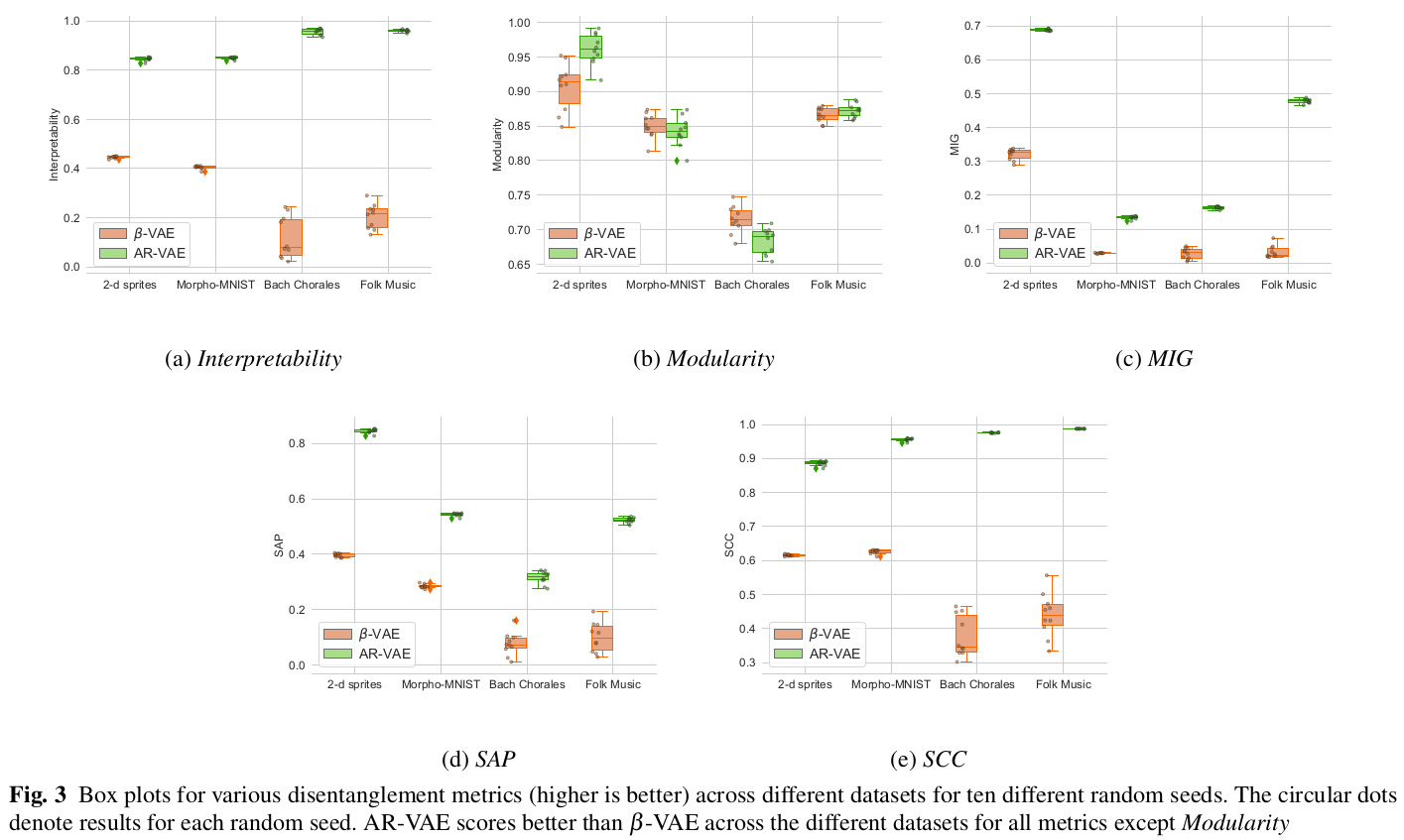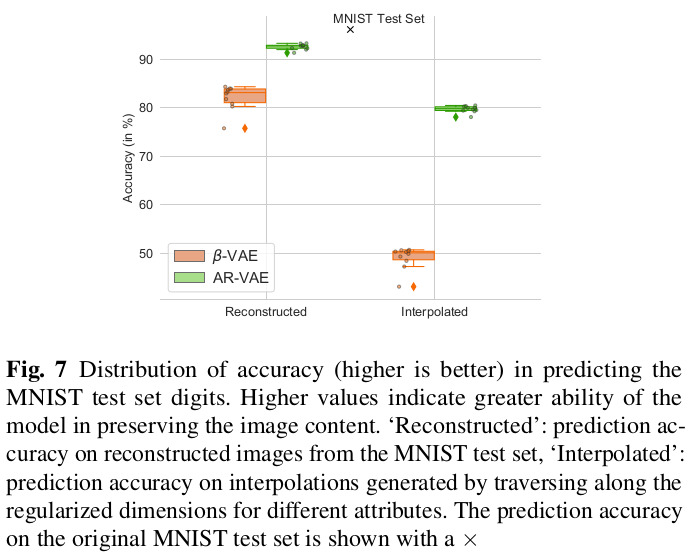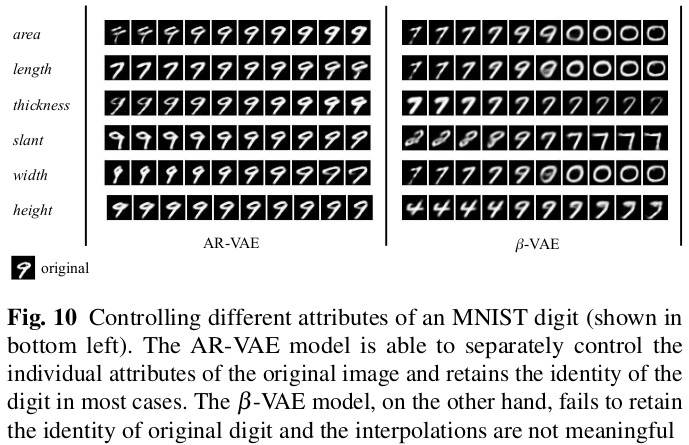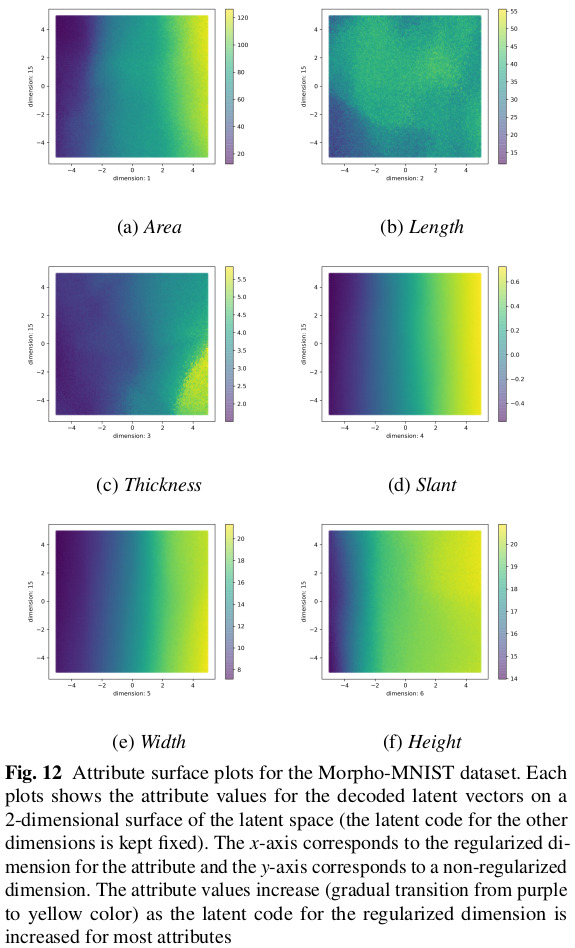# Highlights

• New Attribute-Regularized VAE (AR-VAE) that encodes specific attributes along specific dimensions of the latent space, in a supervised manner;
• Compared to similar methods, AR-VAE as the following advantages:
• has a simple formulation with few hyperparameters,
• works with continuous data attributes, and
• is agnostic to how the attributes are computed/obtained.

# Introduction

The authors introduce a new method to tackle the already well-researched task of disentangling specific factors of the data, called attributes, in a supervised way.

The authors argue that their approach fits a specific type of problem, namely manipulating continuous attributes, for which current methods are not adapted and/or perform poorly:

• Unsupervised disentanglement learning (e.g. $$\beta$$-VAE, FactorVAE1, $$\beta$$-TCVAE2, etc.) requires post-training analysis to identify how attributes are encoded, and consequently how to manipulate them;
• Supervised regularization methods (e.g. Conditional VAEs, Fader networks3, etc.) generally don’t work well for continuous data attributes, like Fader networks that work with binary attributes.

# Methods

The authors simply add what they call an “attribute regularization” loss to a standard $$\beta$$-VAE.

The attribute regularization loss is computed in a three step process. In the steps, attributes are denoted by $$a$$, and $$r$$ indicates a regularized dimension of the latent space.

1. Compute an attribute distance matrix $$D_a \in \mathbb{R}^{m \times m}$$ on a training mini-batch:

$D_a (i,j) = a(\bm{x}_i) - a(\bm{x}_j)$
2. Compute a similar distance matrix $$D_r \in \mathbb{R}^{m \times m}$$ on the dimensions of the latent vectors that you want to regularize:

$D_r (i,j) = z_{i}^{r} - z_{j}^{r}$
3. Compute the regularization loss from both matrices as:

$L_{r,a} = \text{MAE}(\text{tanh}(\delta D_r) - \text{sgn}(D_a))$

where $$\delta$$ is a tunable hyperparameter that decides the spread of the posterior.

The overall loss function for AR-VAE thus becomes:

$L_{\text{AR-VAE}} = L_{\text{recons}} + \beta L_{\text{KLD}} + \gamma \sum_{l=0}^{\mathbb{L}-1} L_{r_{l},a_{l}}$

# Data

The authors point out that most research on disentanglement learning uses image-based datasets. This results in methods being trained, tested and validated on a single domain. To test their method across a wider variety of data, the authors therefore used four datasets across two domains:

• Images
• Morpho-MNIST
• 2-d sprites
• Music
• Measures extracted from the soprano parts of the J.S. Bach Chorales dataset (≈350 chorales)
• Measures extracted (≈20,000) from folk melodies in the Scottish and Irish style

# Results

The full paper contains extensive quantitative and qualitative results on the datasets, so only a very select subset is shown here.# Remarks

• While empirically the attribute regularization seems to work well, theoretically it doesn’t constrain the network from encoding attribute-related information outside of their designated dimensions.